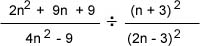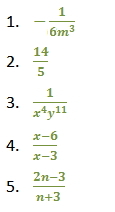Divide Rational Expressions

Divide Rational Expressions

To divide one fraction by a second fraction, multiply the first fraction by the reciprocal of the second fraction.  As with other multiplication problems, factor the numerator and denominator, and look to cancel out common factors before multiplying.

Example - Divide the following expressions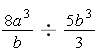Solution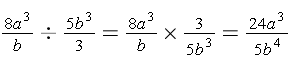Another Example - Divide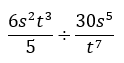As before, multiply by the reciprocal.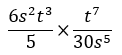Notice there are common factors between a numerator and denominator in this example.  Cancel them by dividing them away.  Remember, to divide variable expressions, subtract their exponents.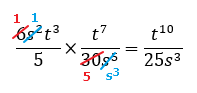Practice

Divide each.

1)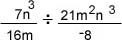2)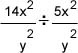3)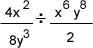4)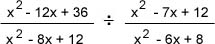5)